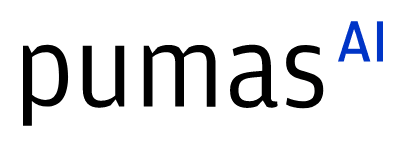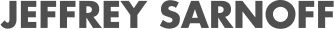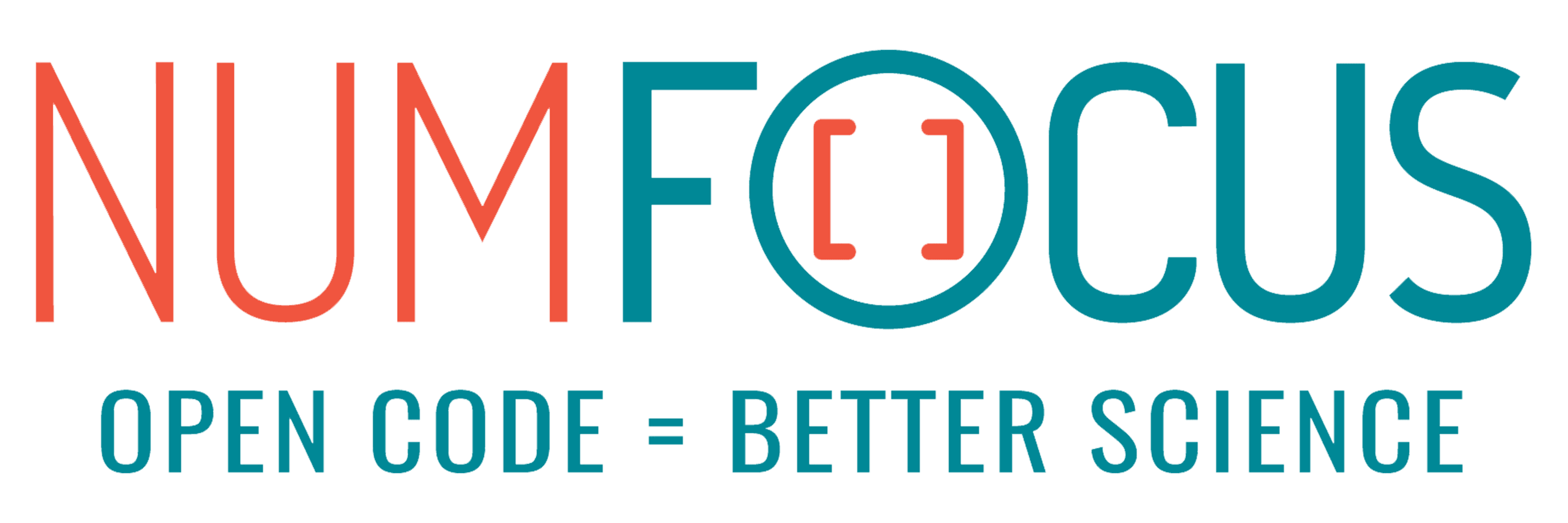# Julia : the unique solution to an optimisation problem

07/27/2023, 2:30 PM2:40 PM UTC
32-124

#### Abstract:

We expose a problem of estimation of multivariate convolutions of gamma random variables, which has very bad numerical properties. This bad numerical behavior literally forced us to use Julia. We describe why Python, R or C++ were not capable of solving our problem and argue that the multiple dispatch paradigm in Julia was the reason we were able to reuse existing code.

#### Description:

The estimation of multivariate generalized Gamma convolutions via their projections into the Laguerre basis is easy to deal with mathematically. However, this deconvolution problem gives a loss function that requires the optimization routine to be:

• Global, since the loss is not convex and has many local minima
• Compiled, since the loss is heavy and cannot be parallelized
• In arbitrary precision, due to combinatorial reasons.

In both Python, R and C++, these three conditions were incompatible and forces us to re-code a full library. However, the ease of code reuse in the Julia ecosystem allowed us to solve our problem using existing libraries.